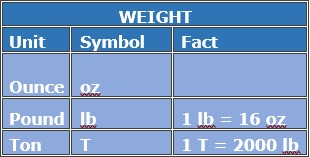# U.S. Customary Unit Conversion with Whole Number Values : Two-Step Conversion

#### Complete Python Prime Pack

9 Courses     2 eBooks

#### Artificial Intelligence & Machine Learning Prime Pack

6 Courses     1 eBooks

#### Java Prime Pack

9 Courses     2 eBooks1 mile = 1,760 yards = 5,280 feet = 63,360 inches1 gallons = 4 quarts = 8 pints = 16 cups1 ton = 2000 pounds = 32000 ounces

Convert between yards and inches?

7 yd = __in

### Solution

Step 1:

12 in = 1 ft

3 ft = 1 yd

Step 2:

So, 7 yd = 7 × 3 ft = 7 × 3 × 12 in = 252 in

Convert between cups (c) and quarts (qt)?

28 c = __qt

### Solution

Step 1:

2 c = 1 pt

2 pt = 1 qt

4 c = 1 qt; 1 c = $\frac{1}{4}$ qt

Step 2:

So, 28 qt = $28 \times \frac{1}{4}$ qt = 7 qt

Convert between ounces (oz) and tons (T)?

3 T = __oz

### Solution

Step 1:

16 oz = 1 lb

2000 lb = 1 T

Step 2:

So, 3 T = 3 × 2000 lb = 3 × 2000 × 16 oz = 96000 oz